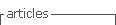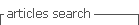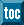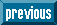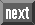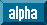## Indicators

•Cited by SciELO
•Access statistics

•Cited by Google
•Similars in SciELO
•Similars in Google

## Print version ISSN 0034-7426

### Rev.colomb.mat. vol.48 no.2 Bogotá July/Dec. 2014

#### https://doi.org/10.15446/recolma.v48n2.54157

Solution of Some Fractional Order Telegraph Equations

Solución de algunas ecuaciones telegráficas de orden fraccional

LEDA GALUÉ1

1Universidad del Zulia, Maracaibo, Venezuela. Email: lgalue@hotmail.com

Abstract

In recent years, there has been a great interest in fractional differential equations due to their frequent appearance in various fields, and their more accurate models of systems under consideration provided by fractional derivatives. In particular, fractional order telegraph equations have been considered and solved for many researchers, using different methods. In this paper we derived the solution of two homogeneous space-time fractional telegraph equations using the generalized differential transform method. The derivatives are considered in Caputo sense and the solutions are given in terms of generalized Mittag-Leffler function and the generalized Wright function. Further, various graphics are included which show the behavior of the solution obtained, and results given earlier by Momani, Odibat and Momani, Yildrim, Garg and Sharma, and Garg et al. are obtained as particular cases of ones our.

Key words: Fractional order telegraph equation, Generalized differential transform method, Caputo fractional derivative, Generalized Mittag-Leffler function, Generalized Wright function.

2000 Mathematics Subject Classification: 35C05, 35C10.

Resumen

Texto completo disponible en PDF

References

 J. Biazar, H. Ebrahimi, and Z. Ayati, 'An Approximation to the Solution of Telegraph Equation by Variational Iteration Method', Numerical Methods for Partial Differential Equations 25, 4 (2009), 797-801.         [ Links ]

 M. Caputo, Elasticita e dissipazione, Zanichelli, Bologna, Italy,         [ Links ] 1969.

 R. C. Cascaval, E. C. Eckstein, C. L. Frota, and J. A. Goldstein, 'Fractional Telegraph Equations', Journal of Mathematical Analysis and Applications 276, 1 (2002), 145-159. http://www.sciencedirect.         [ Links ]com

 S. Das, K. Vishal, P. K. Gupta, and A. Yildirim, 'An Approximate Analytical Solution of Time-Fractional Telegraph Equation', Applied Mathematics and Computation 217, 18 (2011), 7405-7411.         [ Links ]

 L. Debnath, Nonlinear Partial Differential Equations for Scientists and Engineers, Birkhäuser, Boston, USA,         [ Links ] 1997.

 M. Dehghan, S. A. Yousefi, and A. Lotfi, 'The Use of He's Variational Iteration Method for Solving the Telegraph and Fractional Telegraph Equations', Int. J. Numer. Meth. Biomed. Engng. 27, 2 (2011), 219-231. doi: 10.1002/cnm.         [ Links ]1293

 E. C. Eckstein, J. A. Goldstein, and M. Leggas, 'The Mathematics of Suspensions: Kac Walks and Asymptotic Analyticity', Electronic Journal of Differential Equations 3, (1999), 39-50.         [ Links ]

 E. C. Eckstein, M. Leggas, B. Ma, and J. A. Goldstein, Linking Theory and Measurements of Tracer Particle Position in Suspension Flows, 'Proc. ASME FEDSM', (2000), Vol. 251, p.         [ Links ] 1-8.

 M. S. El-Azab and M. El-Gamel, 'A Numerical Algorithm for the Solution of Telegraph Equation', Applied Mathematics and Computation 190, 1 (2007), 757-764.         [ Links ]

 R. Figueiredo, A. O. Chiacchio, and E. C. d. Oliveira, 'Differentiation to Fractional Orders and the Fractional Telegraph Equation', Journal of Mathematical Physics 49, 3 (2008), 1-12. http://dx.doi.org/10.1063/1.2890375         [ Links ]

 N. J. Ford, M. M. Rodrigues, J. Xiao, and Y. Yan, 'Numerical Analysis of a Two-Parameter Fractional Telegraph Equation', Journal of Computational and Applied Mathematics 249, (2013), 95-106.         [ Links ]

 F. Gao and C. Chi, 'Unconditionally Stable Difference Scheme for a One-Space Dimensional Linear Hyperbolic Equation', Applied Mathematics and Computation 187, 2 (2007), 1272-1276.         [ Links ]

 M. Garg, P. Manohar, and S. L. Kalla, 'Generalized Differential Transform Method to Space-Time Fractional Telegraph Equation', International Journal of Differential Equations, (2011), 1-9. doi:10.1155/2011/548982         [ Links ]

 M. Garg and A. Sharma, 'Solution of Space-Time Fractional Telegraph Equation by Adomian Decomposition Method', Journal of Inequalities and Special Functions 2, 1 (2011), 1-7. http://www.ilirias.         [ Links ]com

 G. Hariharan, R. Rajaraman, and M. Mahalakshmi, 'Wavelet Method for a Class of Space and Time Fractional Telegraph Equations', International Journal of Physical Sciences 7, 10 (2012), 1591-1598. http://www.academicjournals.org/IJPS         [ Links ]

 U. Hayat and S. T. Mohyud-Din, 'Homotopy Perturbation Technique for Time-Fractional Telegraph Equations', International Journal of Modern Theoretical Physics 2, 1 (2013), 33-41.         [ Links ]

 F. Huang, 'Analytical Solution for the Time-Fractional Telegraph Equation', Journal of Applied Mathematics, (2009), 1-9. doi:10.1155/2009/890158         [ Links ]

 K. Karimi, A. Niroomand, M. Khaksarfard, and L. Gharacheh, 'On the Numerical Solutions for the Time-Fractional Telegraph Equation', International Journal of Electronics Communication and Computer Engineering 4, 1 (2013), 117-121.         [ Links ]

 D. Kaya, 'A New Approach to the Telegraph Equation: An Application of the Decomposition Method', Bulletin of the Institute of Mathematics, Academia Sinica 28, 1 (2000), 51-57.         [ Links ]

 M. Leggas, Biomedical Engineering, PhD thesis, University of Tennessee Health Sciences Center, Memphis, USA,         [ Links ] 1999.

 F. Mainardi, Fractional Calculus: Some Basic Problems in Continuum and Statistical Mechanics, 'Fractal and Fractional Calculus in Continuum Mechanics', (1997), Springer-Verlag, New York,         [ Links ] USA.

 A. C. Metaxas and R. J. Meredith, Industrial Microwave Heating, Peter Peregrinus, London, UK,         [ Links ] 1993.

 G. M. Mittag-Leffler, 'Sur la nouvelle fonction Eα(x)', Comptes Rendus de l' Académie des Sciences Paris 137, (1903), 554-558.         [ Links ]

 S. Momani, 'Analytic and Approximate Solutions of the Space and Time Fractional Telegraph Equations', Applied Mathematics and Computation 170, 2 (2005), 1126-1134.         [ Links ]

 S. Momani, Z. Odibat, and V. S. Erturk, 'Generalized Differential Transform Method for Solving a Space- and Time -Fractional Diffusion-Wave Equation', Physics Letters A 370, 5-6 (2007), 379-387.         [ Links ]

 Z. Odibat and S. Momani, 'A Generalized Differential Transform Method for Linear Partial Differential Equations of Fractional Order', Applied Mathematics Letters 21, 2 (2008), 194-199. http://www.sciencedirect.         [ Links ]com

 Z. Odibat, S. Momani, and V. S. Erturk, 'Generalized Differential Transform Method: Application to Differential Equations of Fractional Order', Applied Mathematics and Computation 197, 2 (2008), 467-477.         [ Links ]

 E. Orsingher and L. Beghin, 'Time-Fractional Telegraph Equations and Telegraph Processes with Brownian Time', Probability Theory and Related Fields 128, 1 (2004), 141-160.         [ Links ]

 E. Orsingher and X. Zhao, 'The Space-Fractional Telegraph Equation and the Related Fractional Telegraph Process', Chinese Annals of Mathematics Series B 24, 1 (2003), 45-56.         [ Links ]

 I. Podlubny, Fractional Differential Equations, Academic Press, New York, USA,         [ Links ] 1999.

 A. Sevimlican, 'An Approximation to Solution of Space and Time Fractional Telegraph Equations by He's Variational Iteration Method', Mathematical Problems in Engineering, (2010), 1-10. doi:10.1155/2010/290631         [ Links ]

 H. M. Srivastava and P. W. Karlsson, Multiple Gaussian Hypergeometric Series, Jhon Wiley & Sons, New York, USA,         [ Links ] 1985.

 V. A. Vyawahare and P. S. V. Nataraj, Analysis of Fractional-Order Telegraph Model for Neutron Transport in Nuclear Reactor with Slab Geometry, '2013 European Control Conference (ECC)', (2013), Zürich, Switzerland, p. 17-19.         [ Links ]

 A. Wiman, 'Über den Fundamentalsatz in der Teorie der Funktionen Eα(x)', Acta Mathematica 29, 1 (1905), 191-201.         [ Links ]

 Z. Xindong, L. Juan, and W. Leilei, 'An Approximate Analytical Solution for Time-Fractional Telegraph Equation by HPM', Journal of Computational Intelligence and Electronic Systems 1, 1 (2012), 48-53. http://dx.doi.org/10.1166/jcies         [ Links ]

 S. Yakubovich and M. M. Rodrigues, 'Fundamental Solutions of the Fractional Two-Parameter Telegraph Equation', Integral Transforms and Special Functions 23, 7 (2012), 509-519.         [ Links ]

 A. Yildrim, 'He's Homtopy Perturbation Method for Solving the Space and Time Fractional Telegraph Equations', International Journal of Computer Mathematics 87, 13 (2010), 2998-3006.         [ Links ]

(Recibido en mayo de 2014. Aceptado en septiembre de 2014)

Este artículo se puede citar en LaTeX utilizando la siguiente referencia bibliográfica de BibTeX:

` @ARTICLE{RCMv48n2a08,     AUTHOR  = {Galué, Leda},     TITLE   = {{Solution of Some Fractional Order Telegraph Equations}},     JOURNAL = {Revista Colombiana de Matemáticas},     YEAR    = {2014},     volume  = {48},     number  = {2},     pages   = {247--267} } `